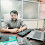# OS Module in Python

8

## import os #os.mkdir("hello") os.chdir("hello") #os.mkdir("hello1") f=open("hello1.txt","w") f.write("hello world") f.close() os.remove("hello1.txt") #os.rmdir("hello1") print(os.getcwd()) #print(os.listdir("c:")) print(os.listdir()) location = 'd:/c/' for file in os.listdir(location):    # if file.endswith(".c"):         print(os.path.join(location, file))

Assignment of os module:-

1)  WAP to count total folder in the computer, drive, max size folder, total image for the particular director, total video on the directory, total python program in the computer.

Solution:-

import os
imgcount=0
txtcount=0
videocount=0
pythonpgrm=0
directory= 'd:/'
maxsize=0
maxfilepath=''
for root, dirs, files in os.walk(directory):

for file in files:
path = os.path.join(root,file)
size = os.stat(path).st_size
if maxsize<size:
maxsize=size
maxfilepath=path

if file.endswith('.png' )or file.endswith('.jpg') :#total image 5825
imgcount = imgcount+1
elif file.endswith('.txt' )or file.endswith('.docx') : # total File 1492
txtcount = txtcount+1
elif file.endswith('.mp3') or file.endswith('.mp4'): #total video 14
videocount=videocount+1
elif file.endswith('.py') : #total python program 238 + compiled file 1754 =1992
pythonpgrm=pythonpgrm+1
#print(a, 'bytes')
print("******In E Drive****** ")
print("Total images are "+str(imgcount))
print("Total text files are "+str(txtcount))
print("Total Video files are "+str(videocount))
print("Total Python Program are "+str(pythonpgrm))
print("Largest file path "+maxfilepath+ " at "+str(maxsize) + " bytes")

Tags

1.Q:- WAP to count total folder in the drive ?
Solution: -
import os
print("The Total Number of Folder's are available in Given Path are : -")
print(len(os.listdir('C:/')))

2.import os
from pathlib import Path

def endswith1(s):
if s.index('.')>=0:
return s[s.index('.'):]
else:
return s

imgcount=0
txtcount=0
entries = Path('d:/')
for entry in entries.iterdir():
print(entry.name)
for entry1 in entry.iterdir():
print(entry1.name)
try:
if endswith1(entry1.name)=='png' or endswith1(entry1.name)=='jpg' or endswith1(entry1.name)=='gif':
imgcount = imgcount+1
elif endswith1(entry1.name)==".txt" or endswith1(entry1.name)==".doc":
txtcount = txtcount+1
except:
pass

print("Total images are "+str(imgcount))
print("Total text files are "+str(txtcount))

3.import os
from pathlib import Path

def endswith1(s):
if s.index('.')>=0:
return s[s.index('.'):]
else:
return s

imgcount=0
txtcount=0
entries = Path('d:/hello')
for entry in entries.iterdir():
print(entry.name)
if os.path.isdir(entry.name):
for entry1 in entry.iterdir():
print(entry1.name)
try:
if endswith1(entry1.name)=='png' or endswith1(entry1.name)=='jpg' or endswith1(entry1.name)=='gif':
imgcount = imgcount+1
elif endswith1(entry1.name)==".txt" or endswith1(entry1.name)==".doc":
txtcount = txtcount+1
except:
pass
else:
if endswith1(entry.name)=='png' or endswith1(entry.name)=='jpg' or endswith1(entry.name)=='gif':
imgcount = imgcount+1
elif endswith1(entry.name)==".txt" or endswith1(entry.name)==".doc":
txtcount = txtcount+1

print("Total images are "+str(imgcount))
print("Total text files are "+str(txtcount))

4.Ankit Saxena

import os
path = input("Enter your directory path: ")
if os.path.isfile(path):
print(f"The given path {path} is a file. please pass only directory path")
else:
all_file = os.listdir(path)
if len(all_file)==0:
print(f"The given path is {path} an empty path")
else:
extension=input("Enter the required files extention .py/ .gif /.docx .txt / .jpg ./.pptx: ")
req_files = []
for x in all_file:
if x.endswith(extension):
req_files.append(x)
if len(req_files)==0:
print(f"There are no {extension} files in the logcation of {path}")
else:
print(f"There are {len(req_files)} files in the location of {path} with an extention of {extension}")
print(f"The files are: {req_files}")

5.#WAP to count total folder in the computer, drive, max size folder, total image for the particular director, total video on the directory, total python program in the computer.
import os
imgcount=0
txtcount=0
videocount=0
pythonpgrm=0
directory= 'c:/'
for root, dirs, files in os.walk(directory):
for file in files:
if file.endswith('.png' )or file.endswith('.jpg') :#total image 5825
imgcount = imgcount+1
elif file.endswith('.txt' )or file.endswith('.docx') : # total File 1492
txtcount = txtcount+1
elif file.endswith('.mp3') or file.endswith('.mp4'): #total video 14
videocount=videocount+1
elif file.endswith('.py') : #total python program 238 + compiled file 1754 =1992
pythonpgrm=pythonpgrm+1
try:
objects = os.listdir("c:")
a=0
name = ""
for item in objects:
size = os.path.getsize(item)
if size > a:
a = size
name = item
print "Largest file is "+name+ " at "+str(a) + " bytes"
print(a, 'bytes')
except WindowsError:
print("\$RECYCLE.BIN not specified")
print("******In C Drive****** ")
print("Total images are "+str(imgcount))
print("Total text files are "+str(txtcount))
print("Total Video files are "+str(videocount))
print("Total Python Program are "+str(pythonpgrm))

6.#ABHISHEK SINGH
#Finding Image/Video/Python Files based on choice
import os
def extension(s):
s1=""
for i in range(len(s)-1,0,-1):
if s[i]=='.':
break
for j in range(i,len(s)):
s1+=s[j]
return s1
1)Press 1 to view file of specific Drive / Path
2)Press 2 to view ALL FILES of your Computer
3)Press any other key/digit to EXIT''')
if command==1:
end=1 #i.e. below outer for loop will only run once in range(0,1) since only single Drive is to be checked
Drive=input("Enter Drive/Path: ")
if ':' not in Drive:
Drive+=':'
elif command==2:
end=24
else:
end=0 #i.e. below outer for loop will NOT run in range(0,0), i.e. Program will NO ACTION takes place.
for d in range(0,end):
if command==2:
Drive=chr(ord("A")+d)+":"
image_list=[]
video_list=[]
python_list=[]
image_count=video_count=python_count=0

for root,subdir,file in os.walk(Drive):
for f in file:
PATH=os.path.join(root,f)
if extension(PATH) in [".jpg",".jpeg",".png",".gif",".tiff",".psd",".eps",".ai"]:
image_count+=1
image_list.append(PATH)
elif extension(PATH) in [".yuv",".wmv",".webm",".vob",".viv",".svi",".roq",".rmvb",".rm",".nsv",".mxf",".mng",".mkv",".m4v",".gifv",".gif",".flv",".flv",".drc",".avi",".asf",".amv",".3gp",".3g2",".qt",".mov ",".f4b",".f4a",".f4v",".flv ",".mpeg",".mp2",".mp4 ",".ts",".m2ts",".mts ",".ogg",".ogv "]:
video_count+=1
video_list.append(PATH)
elif extension(PATH) in [".py"]:
python_count+=1
python_list.append(PATH)
if(image_count+video_count+python_count>0):
print("Drive: ",Drive,":")
print("IMAGE FILES:-\n",image_list,"\nImage Count=",image_count,"\n","-"*50)
print("VIDEO FILES:-\n",video_list,"\nVideo Count=",video_count,"\n","-"*50)
print("PYTHON FILES:-\n",python_list,"\nPython Count=",python_count,"\n","-"*50)

7.import os
os.path.exists
path=input(" enter the path\n")
isExist = os.path.exists(path)
print(isExist)

imgcount = 0
vidcount = 0
txtcount = 0
for path,subdir,files in os.walk(path):
#for file in subdir:
#print (os.path.join(path,name)) # will print path of directories
for file in files:

if file.endswith('.jpg') or file.endswith('.jpeg') or file.endswith('.png'):
imgcount = imgcount+1
elif file.endswith('.mkv') or file.endswith('.mp4'):
vidcount=vidcount+1
elif file.endswith('.txt' )or file.endswith('.docx') : # total File 1492
txtcount = txtcount+1

#print('Size: '+str(max_size)+' bytes')
print("Total images are "+str(imgcount))
print("Total video files are "+str(vidcount))
print("Total text are "+str(txtcount))

8.import os
os.path.exists
path=input(" enter the path\n")
isExist = os.path.exists(path)
print(isExist)

img = 0
vid = 0
txt = 0
for path,subdir,files in os.walk(path):
for file in subdir:
#print (os.path.join(path,name)) # will print path of directories
for file in files:
if file.endswith('.jpg') or file.endswith('.jpeg') or file.endswith('.png'):
img = img+1
elif file.endswith('.mkv') or file.endswith('.mp4'):
vid=vid+1
elif file.endswith('.txt' )or file.endswith('.docx') : # total File 1492
txt = txt+1

print("Total images are "+str(img))
print("Total video files are "+str(vid))
print("Total text are "+str(txt))